# Fitness Function

The 'fitness function' is a rather complex topic which will be discussed in the upcoming sessions more intensively. For now we will define a simple starting point to start with.

We assume a reactive system, i.e. a system with a fixed set of deterministic rules. Additionally we assume a fitness function giving some external values associated with certain events in the environment. In the wood1 environments this is usually the eating of food.

Furthermore we assume an internal action memory called. This set stores the last n-many actions where. It is assumed that the newest action is located at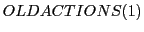. Every time a new action will be selected the old actions will be shifted by 1 to lower positions. If an action receives some fitness valuethen this value will first be attached to the action at, then this value will be 'distributed' to all preceding actions in the.

For the distribution are different strategies possible. The guiding principle should be to enable a 'coherence' between those actions which can contribute to a certain path.

In the case of the maze-learning of rats with a fixed starting point and a highly static environment such a strategy can work quite well. As far as the conditions are becoming more variable (different goals, different starting positions) this mechanism can become to 'weak' to identify a path sufficiently well.

We assume for the length n of thean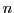at least as long as the smallest path from a start position to a goal (in the TOLMAN1 experiment this would be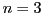).

For the distribution we assume a 'distribution' as follows: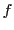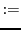(11.1)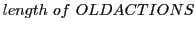(11.2)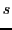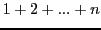(11.3)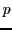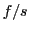(11.4)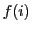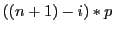(11.5)

Example: Assuming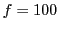,we have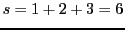,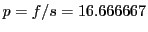and we will have a distribution as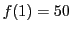,, and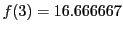. With a normalization bywe can fix the values like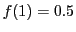,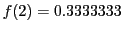, and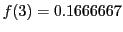.

Because rules can already have some fitness value we have to add the new values to the old ones.

Another aspect is that the fitness values can grow infinitely high and thereby some rules can become 'isolated' from the supporting other rules. To minimize this effect one can introduce a maximum value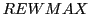and stop the growth above this maximum. This supports a growing similarity between all rules of a certain path. This works as long as there are only a few paths as a subset of all possible paths which lead successfully to a goal state.

We will implement this simple strategy in the first example of a reactive deterministic system and will investigate the pros and cons.

Gerd Doeben-Henisch 2013-01-14